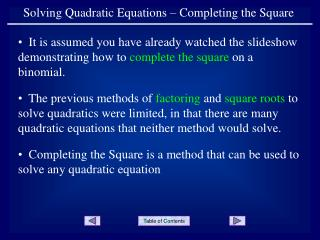DownloadDownload PresentationSolving Quadratic Equations – Completing the Square

# Solving Quadratic Equations – Completing the Square

Télécharger la présentation## Solving Quadratic Equations – Completing the Square

- - - - - - - - - - - - - - - - - - - - - - - - - - - E N D - - - - - - - - - - - - - - - - - - - - - - - - - - -
##### Presentation Transcript

1. Solving Quadratic Equations – Completing the Square • It is assumed you have already watched the slideshow demonstrating how to complete the square on a binomial. • The previous methods of factoring and square roots to solve quadratics were limited, in that there are many quadratic equations that neither method would solve. • Completing the Square is a method that can be used to solve any quadratic equation

2. Write the equation in the form Solving a Quadratic Equation by Completing the Square Complete the square Factor the perfect square trinomial Solve using the square root method

3. Example 1: Solve the equation. Note that the equation is already in the proper form. Complete the square. Add this value to both sides of the equation.

4. Factor Complete the problem using the square root method.

5. Example 2: Solve the equation. Write the equation in the proper form. Complete the square. Be sure to add the same value to both sides of the equation.

6. Factor Complete the problem using the square root method.

7. Example 3: Solve the equation. Write the equation in the proper form. Complete the square and add to both sides. Simplify

8. Factor Complete the problem using the square root method.

9. Use the common denominator to combine the fractions.

10. Example 4: Solve the equation. Write the equation in the proper form. Divide by 3 which is the coefficient of the quadratic term. To be able to complete the square, the coefficient of the quadratic term must be 1.

11. Complete the square. Factor Complete the problem using the square root method.

12. Example 5: Solve the equation. Write the equation in the proper form. Complete the square. Factor

13. Complete the problem using the square root method.

14. END OF PRESENTATION Click to rerun the slideshow.